# Circular trap with integration by parts

## Circular trap

When using integration by parts a second time, make sure you don't choose as the part to integrate the thing you got by differentiating the part to differentiate from the original product. Otherwise, you get in a circular trap and don't get any new information. The most typical application of integration by parts a second time is if you choose to differentiate again the expression that you already obtained through differentiation the first time.

## Formal explanation of circular trap

### Explanation using function notation

Consider an integration of the form:$\int F(x) g(x) \, dx$

where$G$ is an antiderivative for$g$. Then:$\int F(x) g(x) \, dx = F(x)G(x) - \int F'(x)G(x) \, dx$

Suppose that, for the new integral, we choose$G$ as the part to differentiate and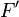$F'$ as the part to integrate. The expression then simplifies to:$\int F(x) g(x) \, dx = F(x)G(x) - [G(x)F(x) - \int g(x)F(x) \, dx]$

Simplifying, we get:$\int F(x) g(x) \, dx = \int F(x)g(x) \, dx$

In other words, we ended up with the original expression, and obtained no new information in the process. Another way of saying this is that we went in circles, i.e., went back along the path we came from, hence did not make any progress.

### Explanation using dependent-independent variable notation

Consider an integration of the form:$\int u \frac{dv}{dx} \, dx$

Using integration by parts, write this as: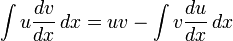$\int u \frac{dv}{dx} \, dx = uv - \int v \frac{du}{dx} \, dx$

Now, let's say that, for the right side integral, we pick our "new"$u$ to be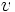$v$ and our "new"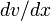$dv/dx$ to be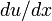$du/dx$. Then, our "new"$v$ is the "old"$u$ and we get: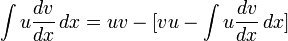$\int u \frac{dv}{dx} \, dx = uv - [vu - \int u \frac{dv}{dx} \, dx]$

Simplifying, we get: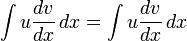$\int u \frac{dv}{dx} \, dx = \int u \frac{dv}{dx} \, dx$

In other words, we ended up with the original expression, and obtained no new information in the process. Another way of saying this is that we went in circles, i.e., went back along the path we came from, hence did not make any progress.

## Using the circular trap to illustrate the intractability of certain problems

### Product of polynomial and trigonometric that integrates to something involving logarithms

Consider the integration problem:$\! int x \tan x \, dx$

If we use the ILATE precedence rule, then, we should take$\tan x$ as the part to integrate and$x$ as the part to differentiate. Using the integration of the tan function to be$-\ln|\cos x$, we get: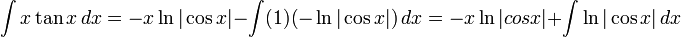$\! \int x \tan x \, dx = -x \ln |\cos x| - \int (1) (-\ln |\cos x|) \, dx = -x\ln|cos x| + \int \ln|\cos x| \, dx$

Now, we reconsider the ILATE precedence rule to integrate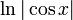$\ln|\cos x|$. The function comes closest to being a logarithmic function, hence ILATE would suggest that we rewrite it as$\ln|\cos x|$ times 1, picking 1 as the part to integrate. However, we immediately see that making this choice would fall into the circular trap.

Since there is no other reasonable choice to make, this problem is not tractable using integration by parts and the standard function integrations.

Note, however, that we did manage to establish an equivalence of integration problems: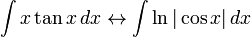$\int x \tan x \, dx \leftrightarrow \int \ln|\cos x| \, dx$

### Product of inverse trigonometric or logarithmic function and rational function with integral that is inverse trigonometric or logarithmic

Consider an integral of the form:$\int \frac{\arctan x}{x} \, dx$

ILATE suggests that we take$\arctan$ (the inverse trigonometric function) as the part to differentiate and$1/x$ (the algebraic function) as the part to integrate. We get:$\int \frac{\arctan x}{x} \, dx = (\arctan x)(\ln |x|) - \int \frac{\ln |x| \, dx}{x^2 + 1}$

Now, ILATE suggests that for the integral on the right side, we take$\ln |x|$ (the logarithmic function) as the part to differentiate) and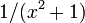$1/(x^2 + 1)$ as the part to integrate. However, we immediately see that making this choice would fall into the circular trap.

In fact, there is no other reasonable choice to make, so this integral cannot be done using the standard function integrations. Note, however, that we did manage to establish an equivalence of integration problems:$\int \frac{\arctan x}{x} \, dx \leftrightarrow \int \frac{\ln |x|}{x^2 + 1} \, dx$